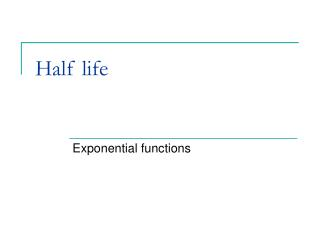DownloadDownload PresentationHalf life

Half life

Télécharger la présentationHalf life

- - - - - - - - - - - - - - - - - - - - - - - - - - - E N D - - - - - - - - - - - - - - - - - - - - - - - - - - -
Presentation Transcript

1. Half life Exponential functions

2. Half Life [ Definition : Half-life- the time it takes for one-half of the atoms of an unstable element or nuclide to decay radioactively into another element or nuclide. ]

3. Radium has a half life of 1600 years. If A0 = 8 grams, show that the decay function for the mass remaining after n years is given by Use this function to find the mass left after (i) 40 years (ii) 200 years

4. A radioactive element has a half life of only 20 years. Write down a decay function for 40 grams of the material after n years. a) Calculate the mass left after 15 years. b) Calculate the mass left after 45 years.

5. The half life of C14 the radioactive form of carbon is 5730 years. If 5 gram of carbon is initially present, construct a decay function that models the amount left after n years. How much will be left after 10000 years?

6. The table shows some materials, their half lives and their area of application. Use this table to answer the questions on the next slide.

7. For each material set up a decay function for 50 grams of the material. Use these decay functions to answer the following questions. How much Iodine – 123, is left after 1 day? How much Cobalt -60, is left after 8 years? How much Plutonium-239, is left after 1000 years?